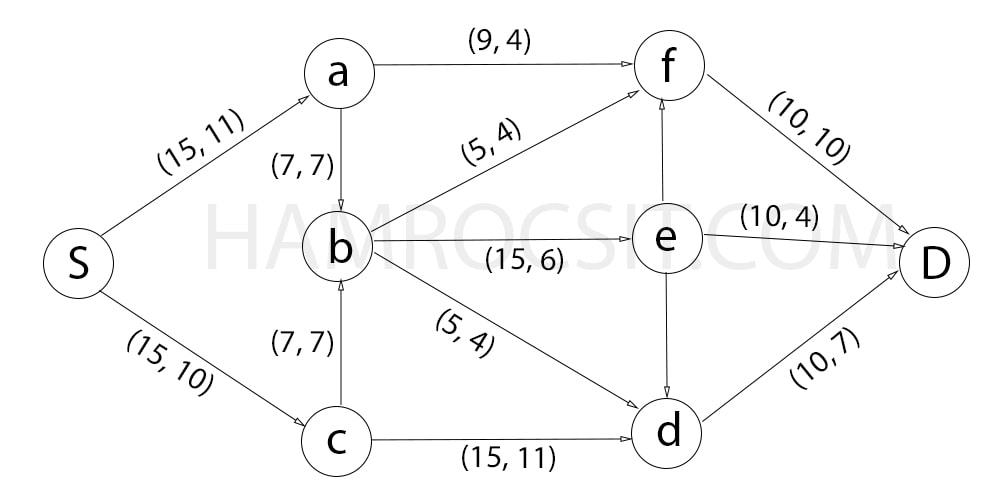### Exam Year

Tribhuvan University

Institute of Science and Technology

2075

Bachelor Level / second-semester / Science

Computer Science and Information Technology( CSC160 )

Discrete Structure

Full Marks: 60 + 20 + 20

Pass Marks: 24 + 8 + 8

Time: 3 Hours

Candidates are required to give their answers in their own words as far as practicable.

The figures in the margin indicate full marks.

Group A

Attempt any two questions:(2 x 10 = 20)

1

What is S-D cut? For the following network flow find the maximal flow from S to D.2

Consider a set U = {1, 2, 3, 4, 5, 6, 7, 8, 9, 10}. What will be the computer representation for set containing the numbers which are multiple of 3 not exceeding 6? Describe injective, Surjective and bijective function with example.

3

Compute the following values.

a. 3 mod 4          b. 7 mod 5           c. -5 mod 3           d. 11 mod 5          e. -8  mod 6

Write down the recursive algorithm to find the value of bn and prove its correctness using induction.

Group B

Attempt any eight questions:(8 x 5 = 40)

4

Solve the recurrence relation an = 5an-1 – 6an-2 with initial conditions a= 1 and a= 2.

5

Find the value of x such that x = 1 (mod 5) and x = 2 (mod 7) using Chinese remainder theorem.

6

Prove that 5n-1 is divisible by 4 using mathematical induction.

7

Let A = “Aldo is Italian” and B = “Bob is English”. Formalize the following sentences in proposition.

1. Aldo isn’t Italian.
2. Aldo is Italian while Bob is English.
3. If Aldo is Italian then Bob Bob is not English.
4. Aldo is Italian or if Aldo isn’t Italian then Bob is English.
5. Either Aldo is Italian and Bob is English, or neither Aldo is Italian nor Bob is English.
8

Define Eular path and Hamilton path with examples. Draw the Hasse diagram for the divisible relation on the set { 1, 2, 5, 8, 16, 32} and find the maximal, minimal, greatest and least element if exist.

9

What does primality testing means? Describe how Fermat’s Little Theorem tests for a prime number with suitable example.

10

List any two applications of conditional probability. You have 9 families you would like to invite to a wedding. Unfortunately, you can only invite 6 families. How many different sets of invitations could you write?

11

Define spanning tree and minimum spanning tree. Mention the conditions for two graphs for being isomorphic with an example.

12

Prove that the product xy is odd if and only if both x and y are odd integers .